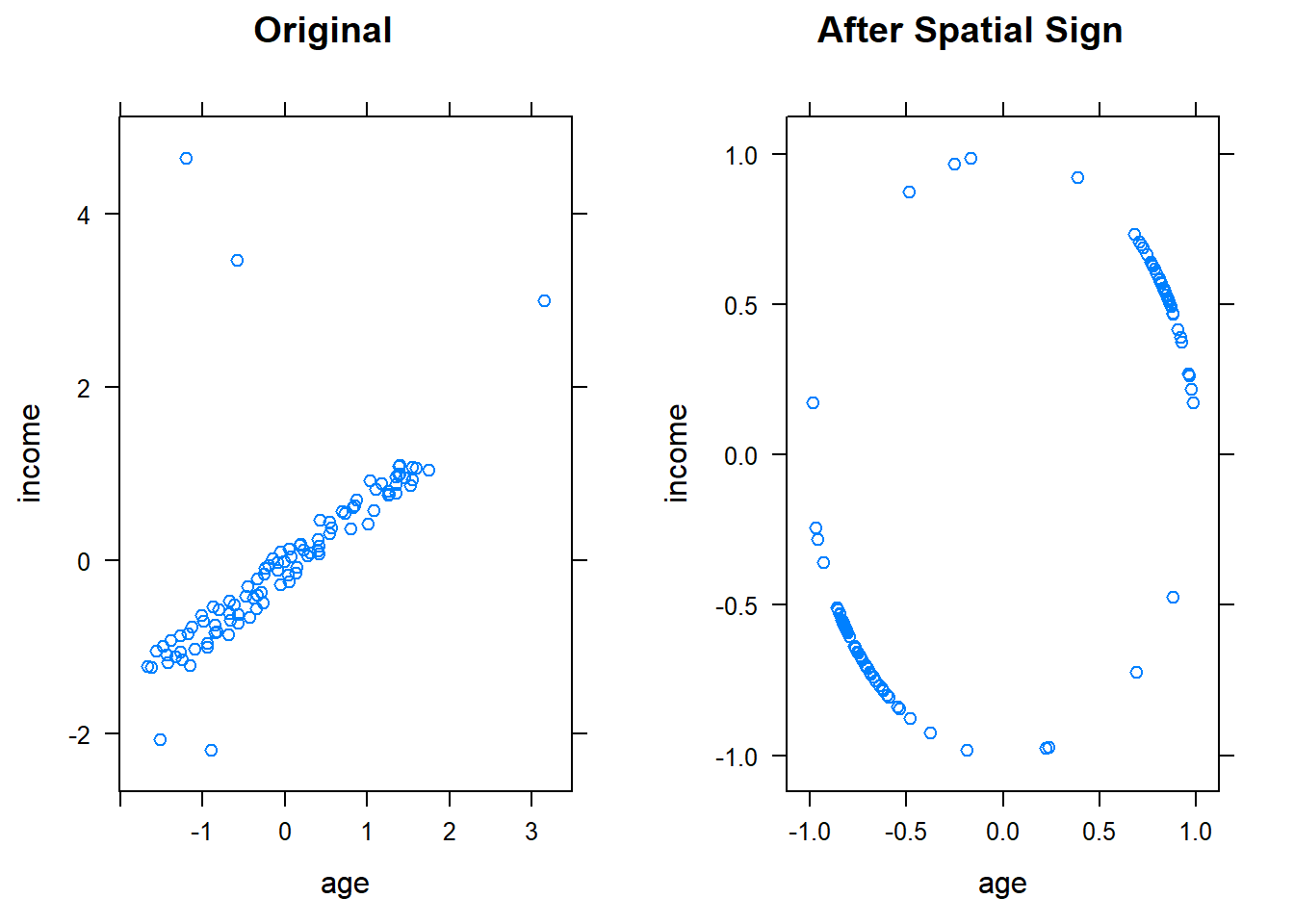## Resolve Outliers

Even under certain assumptions we can statistically define outliers, it can be hard to define in some situations. Some models are resistant to outliers (such as tree-based models and support vector machines). If a model is sensitive to outliers (like linear regression and logistic regression), we can use spatial sign transformation to minimize the problem. This procedure projects the predictor values onto a multidimensional sphere. This has the effect of making all the samples the same distance from the center of the sphere. (Factors are not allowed.)

It is important to center and scale the predictor data prior to using this transformation. This does not remove outliers - it is just a preprocessing method which brings the outliers towards the majority of the data.

Once again, caret comes to the rescue. (I am seriously considering spending the next year just learning everything the caret package can do!)

First, create some data - yes, similar to the same one I have been using but with a few changes to bring out the outliers for this exercise.

#Make up some data
income <- sample(seq(50000, 150000, by = 500), 95)
age <- income/2000-10
noise <- round(runif(95) * 10, 0)
age <- age + noise
income <- c(income, 10000, 15000, 300000, 250000, 230000)
age <- c(age, 30, 20, 25, 35, 95)
myData <- data.frame(income, age)
myData\$education <- as.factor(sample(c("High School", "Bachelor", "Master", "Doctor"), 100, replace = TRUE, prob =c(0.7, 0.15, 0.12, 0.03) ))

Use spatialSign() in caret to conduct spatial sign:

myTrans <- preProcess(myData[, c("income", "age")], method=c("center", "scale"))
myTransformed <- predict(myTrans, myData[, c("income", "age")])
myTransformed2 <- spatialSign(myTransformed)
myTransformed2 <- as.data.frame(myTransformed2)
head(myTransformed2, 10)
##        income        age
## 1  -0.7029692 -0.7112203
## 2  -0.5498582 -0.8352580
## 3  -0.5807168 -0.8141057
## 4   0.5542554  0.8323467
## 5  -0.6733373 -0.7393355
## 6   0.5549489  0.8318844
## 7   0.5396606  0.8418827
## 8  -0.5148900 -0.8572563
## 9   0.5444314  0.8388053
## 10 -0.9714357  0.2373030
p1 <- xyplot(income ~ age, data = myTransformed, main="Original")
p2 <- xyplot(income ~ age, data = myTransformed2, main="After Spatial Sign")
grid.arrange(p1, p2, ncol = 2)